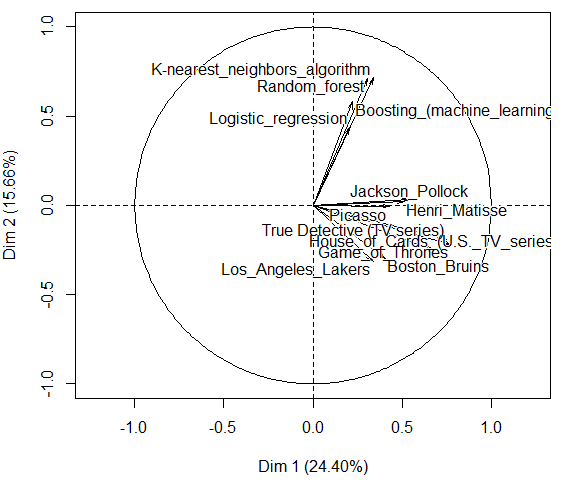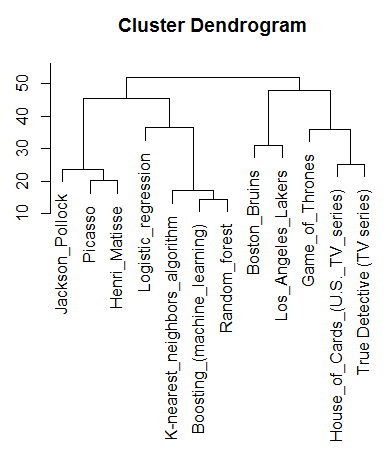Want to share your content on R-bloggers? click here if you have a blog, or here if you don't.

Another popular application of classification techniques is on texmining (see e.g. an old post on French president speaches). Consider the following example,  inspired by Nobert Ryciak’s post, with 12 wikipedia pages, on various topics,

```> library(tm)
> library(stringi)
> library(proxy)
> titles = c("Boosting_(machine_learning)",
+            "Random_forest",
+            "K-nearest_neighbors_algorithm",
+            "Logistic_regression",
+            "Boston_Bruins",
+            "Los_Angeles_Lakers",
+            "Game_of_Thrones",
+            "House_of_Cards_(U.S._TV_series)",
+            "True Detective (TV series)",
+            "Picasso",
+            "Henri_Matisse",
+            "Jackson_Pollock")
> articles = character(length(titles))
> for (i in 1:length(titles)) {
+   articles[i] = stri_flatten(readLines(stri_paste(wiki, titles[i])), col = " ")
+ }```

Here, we store all the contents of the pages in a corpus (from the text mining package).

`> docs = Corpus(VectorSource(articles))`

This is what we have in that corpus

```> a = stri_flatten(readLines(stri_paste(wiki, titles)), col = " ")
> a = Corpus(VectorSource(a))
> a[]

Thoughts on Hypothesis Boosting</i></a>, Unpublished manuscript (Machine Learning class project, December 1988)</span></li> <li id="cite_note-4"><span class="mw-cite-backlink"><b><a href="#cite_ref-4">^</a></b></span> <span class="reference-text"><cite class="citation journal"><a href="/wiki/Michael_Kearns" title="Michael Kearns">Michael Kearns</a>; <a href="/wiki/Leslie_Valiant" title="Leslie Valiant">Leslie Valiant</a> (1989). <a rel="nofollow" class="external text" href="http://dl.acm.org/citation.cfm?id=73049">"Crytographic limitations on learning Boolean formulae and finite automata"</a>. <i>Symposium on T```

This is because we read an html page.

```> a = tm_map(a, function(x)
> a = tm_map(a, function(x) stri_replace_all_fixed(x, "t", " "))
> a = tm_map(a, PlainTextDocument)
> a = tm_map(a, stripWhitespace)
> a = tm_map(a, removeWords, stopwords("english"))
> a = tm_map(a, removePunctuation)
> a = tm_map(a, tolower)
> a

can  set  weak learners create  single strong learner  a weak learner  defined    classifier    slightly correlated   true classification  can label examples better  random guessing in contrast  strong learner   classifier   arbitrarily wellcorrelated   true classification robert ```

Now we have the text of the wikipedia document. What we did was

• replace all “” elements with a space. We do it because there are not a part of text document but in general a html code.
• replace all “/t” with a space.
• convert previous result (returned type was “string”) to “PlainTextDocument”, so that we can apply the other functions from tm package, which require this type of argument.
• remove extra whitespaces from the documents.
• remove punctuation marks.
• remove from the documents words which we find redundant for text mining (e.g. pronouns, conjunctions). We set this words as stopwords(“english”) which is a built-in list for English language (this argument is passed to the function removeWords.
• transform characters to lower case.

Now we can do it on the entire corpus

```> docs2 = tm_map(docs, function(x) stri_replace_all_regex(x, "<.+?>", " "))
> docs3 = tm_map(docs2, function(x) stri_replace_all_fixed(x, "t", " "))
> docs4 = tm_map(docs3, PlainTextDocument)
> docs5 = tm_map(docs4, stripWhitespace)
> docs6 = tm_map(docs5, removeWords, stopwords("english"))
> docs7 = tm_map(docs6, removePunctuation)
> docs8 = tm_map(docs7, tolower)```

Now, we simply count words in each page,

```> dtm <- DocumentTermMatrix(docs8)
> dtm2 <- as.matrix(dtm)
> dim(dtm2)
 12 13683
> frequency <- colSums(dtm2)
> frequency <- sort(frequency, decreasing=TRUE)
> mots=frequency[frequency>20]
> s=dtm2[1,which(colnames(dtm2) %in% names(mots))]
> for(i in 2:nrow(dtm2)) s=cbind(s,dtm2[i,which(colnames(dtm2) %in% names(mots))])
> colnames(s)=titles```

Once we have that dataset, we can use a PCA to visualise the ‘variables’ i.e. the pages

```> library(FactoMineR)
> PCA(s)```We can also use non-supervised classification to group pages. But first, let us normalize the dataset

`> s0=s/apply(s,1,sd)`

Then, we can run a cluster dendrogram, using the Ward distance

```> h <- hclust(dist(t(s0)), method = "ward")
> plot(h, labels = titles, sub = "")```Groups are consistent with intuition: painters are in the same cluster, as well as TV series, sports teams, and statistical techniques.